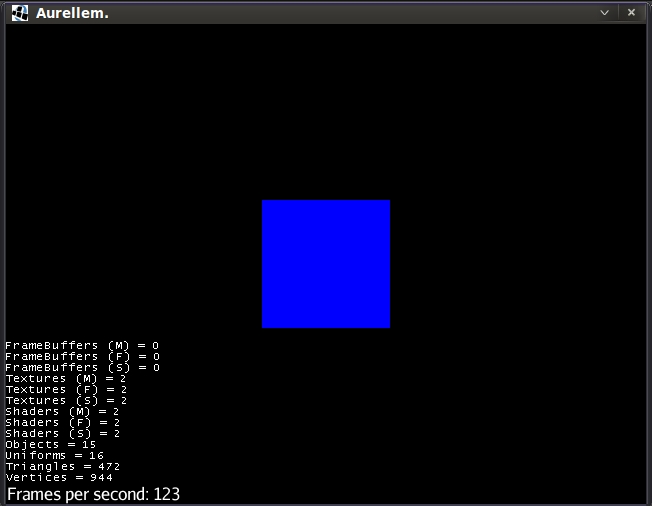# aurellem ☉

[TABLE-OF-CONTENTS]

Here are the jMonkeyEngine "Hello" programs translated to clojure.

## 1 Hello Simple App

Here is the hello world example for jme3 in clojure. It's a more or less direct translation from the java source here.

Of note is the fact that since we don't have access to the `AssetManager` via extending `SimpleApplication`, we have to build one ourselves.

```(ns hello.hello-simple-app
(:use cortex.world)
(:import com.jme3.math.Vector3f)
(:import com.jme3.material.Material)
(:import com.jme3.scene.Geometry)
(:import com.jme3.math.ColorRGBA)
(:import com.jme3.app.SimpleApplication)
(:import com.jme3.scene.shape.Box))

(def cube (Box. Vector3f/ZERO 1 1 1))

(def geom (Geometry. "Box" cube))

(.setColor mat "Color" ColorRGBA/Blue)

(.setMaterial geom mat)

(defn simple-app []
(doto
(proxy [SimpleApplication] []
(simpleInitApp
[]
;; Don't take control of the mouse
(org.lwjgl.input.Mouse/setGrabbed false)
(.attachChild (.getRootNode this) geom)))
;; don't show a menu to change options.
(.setShowSettings false)
(.setPauseOnLostFocus false)
(.setSettings *app-settings*)))
```

Running this program will begin a new jMonkeyEngine game which displays a single blue cube.

```(.start (hello.hello-simple-app/simple-app))
```Figure 1: the simplest JME game.

## 2 Simpler HelloSimpleApp

```(ns hello.hello-simpler-app
(:use cortex.world)
(:use cortex.util)
(:import com.jme3.math.ColorRGBA)
(:import com.jme3.scene.Node))

(defn simpler-world
"The jMonkeyEngine3 Hello World program.  Displays a blue 3D cube in
a basic 3D world."
[]
(world (doto (Node.)
(.attachChild
(box 1 1 1
:color ColorRGBA/Blue :physical? false)))
{} no-op no-op))
```

## 3 Hello Loop

```(ns hello.loop
(:use cortex.world)
(:use cortex.util)
(:import com.jme3.math.ColorRGBA)
(:import com.jme3.scene.Node))

(defn blue-cube []
(box 1 1 1
:color ColorRGBA/Blue
:texture false
:name "blue-cube"
:physical? false))

(defn blue-cube-game []
(let [cube (blue-cube)
root (doto (Node.) (.attachChild cube))]
(world root
{}
no-op
(fn [game tpf]
(.rotate cube 0.0 (* 2 tpf) 0.0)))))
```

Created: 2015-04-19 Sun 07:04

Emacs 24.4.1 (Org mode 8.3beta)

Validate